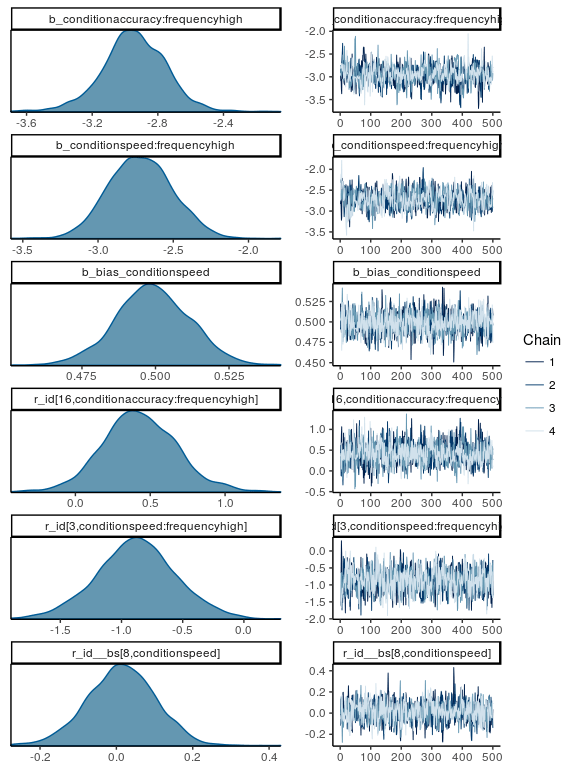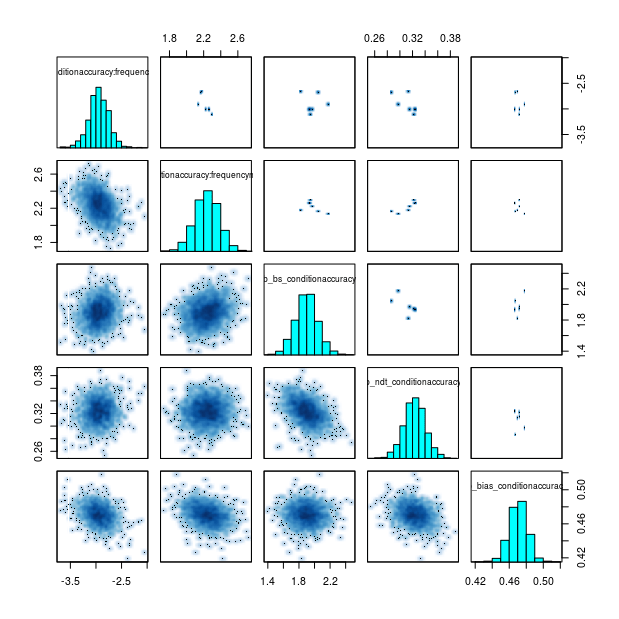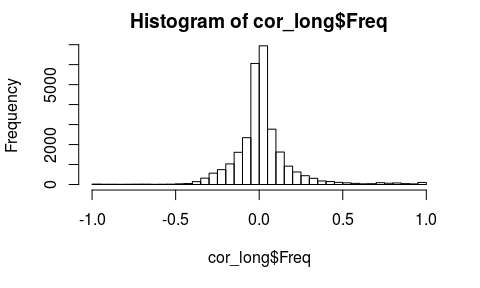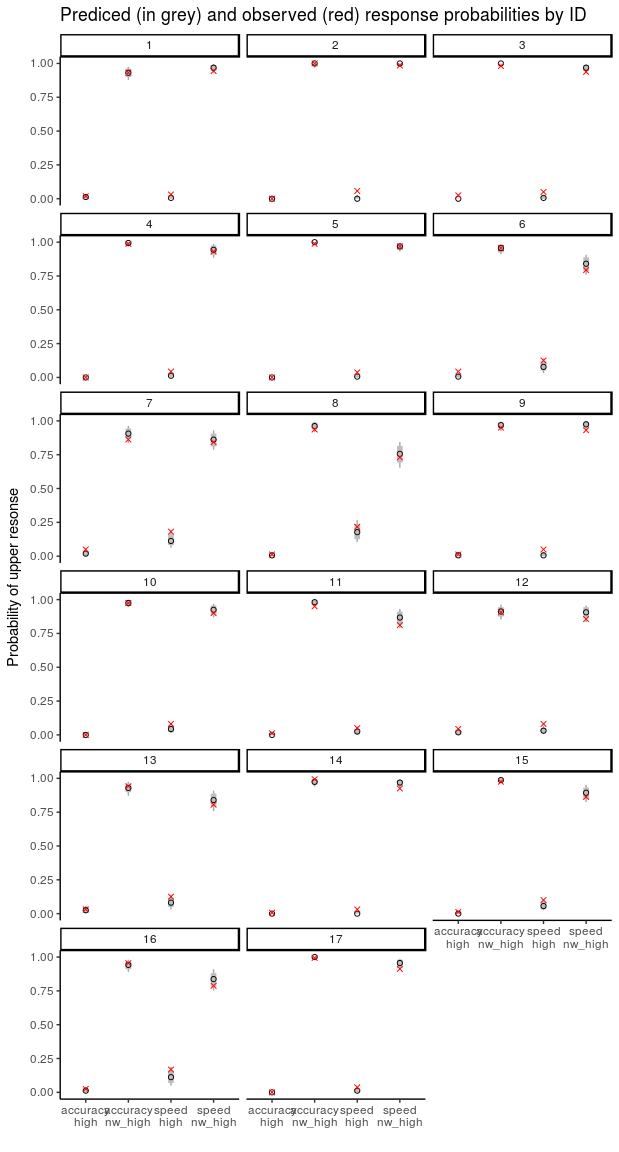# Diffusion/Wiener Model Analysis with brms – Part II: Model Diagnostics and Model Fit

This is the considerably belated second part of my blog series on fitting diffusion models (or better, the 4-parameter Wiener model) with `brms`. The first part discusses how to set up the data and model. This second part is concerned with perhaps the most important steps in each model based data analysis, model diagnostics and the assessment of model fit. Note, the code in the part is completely self sufficient and can be run without running the code of part I.

### Setup

At first, we load quite a few packages that we will need down the way. Obviously `brms`, but also some of the packages from the `tidyverse` (i.e., `dplyr`, `tidyr`, `tibble`, and `ggplot2`). It took me a little time to jump on the `tidyverse` bandwagon, but now that I use it more and more I cannot deny its utility. If your data can be made ‘tidy’, the coherent set of tools offered by the `tidyverse` make many seemingly complicated tasks pretty easy. A few examples of this will be shown below. If you need more introduction, I highly recommend the awesome ‘R for Data Science’ book by Grolemund and Wickham, which they made available for free! We also need `gridExtra` for combining plots and `DescTools` for the concordance correlation coefficient CCC used below.

```library("brms")
library("dplyr")
library("tidyr")
library("tibble")    # for rownames_to_column
library("ggplot2")
library("gridExtra") # for grid.arrange
library("DescTools") # for CCC```

As in part I, we need package `rtdists` for the data.

```data(speed_acc, package = "rtdists")
speed_acc <- droplevels(speed_acc[!speed_acc\$censor,]) # remove extreme RTs
speed_acc <- droplevels(speed_acc[ speed_acc\$frequency %in%
c("high", "nw_high"),])
speed_acc\$response2 <- as.numeric(speed_acc\$response)-1```

I have uploaded the binary R data file containing the fitted model object as well as the generated posterior predictive distributions to github, from which we can download them directly into `R`. Note that I needed to go the way via a temporary folder. If there is a way without that I would be happy to learn about it.

```tmp <- tempdir()
file.path(tmp, "brms_wiener_example_fit.rda"))
file.path(tmp, "brms_wiener_example_predictions.rda"))

### Model Diagnostics

We already know from part I that there are a few divergent transitions. If this were a real analysis we therefore would not be satisfied with the current fit and try to rerun `brm` with an increased `adapt_delta` with the hope that this removes the divergent transitions. The Stan warning guidelines clearly state that “the validity of the estimates is not guaranteed if there are post-warmup divergences”. However, it is unclear what the actual impact of the small number of divergent transitions (< 10) observed here is on the posterior. Also, it is unclear what one can do if `adapt_delta` cannot be increased anymore and the model also cannot be reparameterized. Should all fits with any divergent transitions be completely disregarded? I hope the Stan team provides more guidelines to such questions in the future.

Coming back to our fit, as a first step in our model diagnostics we check the R-hat statistic as well as the number of effective samples. Specifically, we look at the parameters with the highest R² and lowest number of effective samples.

```tail(sort(rstan::summary(fit_wiener\$fit)\$summary[,"Rhat"]))
#                      sd_id__conditionaccuracy:frequencyhigh
#                                                        1.00
#                              r_id__bs[15,conditionaccuracy]
#                                                        1.00
#                                    b_bias_conditionaccuracy
#                                                        1.00
# cor_id__conditionspeed:frequencyhigh__ndt_conditionaccuracy
#                                                        1.00
#                                   sd_id__ndt_conditionspeed
#                                                        1.00
#  cor_id__conditionspeed:frequencynw_high__bs_conditionspeed
#                                                        1.01
#                                     lp__
#                                      462
#        b_conditionaccuracy:frequencyhigh
#                                      588
#                sd_id__ndt_conditionspeed
#                                      601
#      sd_id__conditionspeed:frequencyhigh
#                                      646
#           b_conditionspeed:frequencyhigh
#                                      695
# r_id[12,conditionaccuracy:frequencyhigh]
#                                      712```

Both are unproblematic (i.e., `R-hat` < 1.05 and `n_eff` > 100) and suggest that the sampler has converged on the stationary distribution. If anyone has a similar oneliner to return the number of divergent transitions, I would be happy to learn about it.

We also visually inspect the chain behavior of a few semi-randomly selected parameters.

```pars <- parnames(fit_wiener)
pars_sel <- c(sample(pars[1:10], 3), sample(pars[-(1:10)], 3))
plot(fit_wiener, pars = pars_sel, N = 6,
ask = FALSE, exact_match = TRUE, newpage = TRUE, plot = TRUE)```This visual inspection confirms the earlier conclusion. For all parameters the posteriors look well-behaved and the chains appears to mix well.

Finally, in the literature there are some discussions about parameter trade-offs for the diffusion and related models. These trade-offs supposedly make fitting the diffusion model in a Bayesian setting particularly complicated. To investigate whether fitting the Wiener model with HMC as implemented in `Stan` (i.e., NUTS) also shows this pattern we take a look at the joint posterior of the fixed-effects of the main Wiener parameters for the accuracy condition. For this we use the `stanfit` method of the `pairs` function and set the `condition` to `"divergent__"`. This plots the few divergent transitions above the diagonal and the remaining samples below the diagonal.

`pairs(fit_wiener\$fit, pars = pars[c(1, 3, 5, 7, 9)], condition = "divergent__")`This plot shows some correlations, but nothing too dramatic. HMC appears to sample quite efficiently from the Wiener model.

Next we also take a look at the correlations across all parameters (not only the fixed effects).

```posterior <- as.mcmc(fit_wiener, combine_chains = TRUE)
cor_posterior <- cor(posterior)
cor_posterior[lower.tri(cor_posterior, diag = TRUE)] <- NA
cor_long <- as.data.frame(as.table(cor_posterior))
cor_long <- na.omit(cor_long)
tail(cor_long[order(abs(cor_long\$Freq)),], 10)
#                              Var1                         Var2   Freq
# 43432        b_ndt_conditionspeed  r_id__ndt[1,conditionspeed] -0.980
# 45972 r_id__ndt[4,conditionspeed] r_id__ndt[11,conditionspeed]  0.982
# 46972        b_ndt_conditionspeed r_id__ndt[16,conditionspeed] -0.982
# 44612        b_ndt_conditionspeed  r_id__ndt[6,conditionspeed] -0.983
# 46264        b_ndt_conditionspeed r_id__ndt[13,conditionspeed] -0.983
# 45320        b_ndt_conditionspeed  r_id__ndt[9,conditionspeed] -0.984
# 45556        b_ndt_conditionspeed r_id__ndt[10,conditionspeed] -0.985
# 46736        b_ndt_conditionspeed r_id__ndt[15,conditionspeed] -0.985
# 44140        b_ndt_conditionspeed  r_id__ndt[4,conditionspeed] -0.990
# 45792        b_ndt_conditionspeed r_id__ndt[11,conditionspeed] -0.991```

This table lists the ten largest absolute values of correlations among posteriors for all pairwise combinations of parameters. The value in column `Freq` somewhat unintuitively is the observed  correlation among the posteriors of the two parameters listed in the two previous columns. To create this table I used a trick from SO using `as.table`, which is responsible for labeling the column containing the correlation value `Freq`.

What the table shows is some extreme correlations for the individual-level deviations (the first index in the squared brackets of the parameter names seems to be the participant number). Let us visualize these correlations as well.

```pairs(fit_wiener\$fit, pars =
c("b_ndt_conditionspeed",
"r_id__ndt[11,conditionspeed]",
"r_id__ndt[4,conditionspeed]"),
condition = "divergent__")```This plot shows that some of the individual-level parameters are not well estimated.

However, overall these extreme correlations appear rather rarely.

`hist(cor_long\$Freq, breaks = 40)`Overall the model diagnostics do not show any particularly worrying behavior (with the exception of the divergent transitions). We have learned that a few of the individual-level estimates for some of the parameters are not very trustworthy. However, this does not disqualify the overall fit. The main take away from this fact is that we would need to be careful in interpreting the individual-level estimates. Thus, we assume the fit is okay and continue with the next step of the analysis.

### Assessing Model Fit

We will now investigate the model fit. That is, we will investigate whether the model provides an adequate description of the observed data. We will mostly do so via graphical checks. To do so, we need to prepare the posterior predictive distribution and the data. As a first step, we combine the posterior predictive distributions with the data.

`d_speed_acc <- as_tibble(cbind(speed_acc, as_tibble(t(pred_wiener))))`

Then we calculate three important measures (or test statistics T()) on the individual level for each cell of the design (i.e., combination of `condition` and `frequency` factors):

• Probability of giving an upper boundary response (i.e., respond “nonword”).
• Median RTs for responses to the upper boundary.
• Median RTs for the lower boundary.

We first calculate this for each sample of the posterior predictive distribution. We then summarize these three measures by calculating the median and some additional quantiles across the posterior predictive distribution. We calculate all of this in one step using a somewhat long combination of `dplyr` and `tidyr` magic.

```d_speed_acc_agg <- d_speed_acc %>%
group_by(id, condition, frequency) %>%  # select grouping vars
summarise_at(.vars = vars(starts_with("V")),
funs(prob.upper = mean(. > 0),
medrt.lower = median(abs(.[. < 0]) ),
medrt.upper = median(.[. > 0] )
)) %>%
ungroup %>%
gather("key", "value", -id, -condition, -frequency) %>% # remove grouping vars
separate("key", c("rep", "measure"), sep = "_") %>%
group_by(id, condition, frequency) %>% # select grouping vars
summarise_at(.vars = vars(prob.upper, medrt.lower, medrt.upper),
.funs = funs(median = median(., na.rm = TRUE),
llll = quantile(., probs = 0.01,na.rm = TRUE),
lll = quantile(., probs = 0.025,na.rm = TRUE),
ll = quantile(., probs = 0.1,na.rm = TRUE),
l = quantile(., probs = 0.25,na.rm = TRUE),
h = quantile(., probs = 0.75,na.rm = TRUE),
hh = quantile(., probs = 0.9,na.rm = TRUE),
hhh = quantile(., probs = 0.975,na.rm = TRUE),
hhhh = quantile(., probs = 0.99,na.rm = TRUE)
))```

Next, we calculate the three measures also for the data and combine it with the results from the posterior predictive distribution in one `data.frame` using `left_join`.

```speed_acc_agg <- speed_acc %>%
group_by(id, condition, frequency) %>% # select grouping vars
summarise(prob.upper = mean(response == "nonword"),
medrt.upper = median(rt[response == "nonword"]),
medrt.lower = median(rt[response == "word"])
) %>%
ungroup %>%
left_join(d_speed_acc_agg)```

#### Aggregated Model-Fit

The first important question is whether our model can adequately describe the overall patterns in the data aggregated across participants. For this we simply aggregate the results obtained in the previous step (i.e., the summary results from the posterior predictive distribution as well as the test statistics from the data) using `mean`.

```d_speed_acc_agg2 <- speed_acc_agg %>%
group_by(condition, frequency) %>%
summarise_if(is.numeric, mean, na.rm = TRUE) %>%
ungroup```

We then use these summaries and plot predictions (in grey and black) as well as data (in red) for the three measures. The inner (fat) error bars show the 80% credibility intervals (CIs), the outer (thin) error bars show the 95% CIs. The black circle shows the median of the posterior predictive distributions.

```new_x <- with(d_speed_acc_agg2,
paste(rep(levels(condition), each = 2),
levels(frequency), sep = "\n"))

p1 <- ggplot(d_speed_acc_agg2, aes(x = condition:frequency)) +
geom_linerange(aes(ymin =  prob.upper_lll, ymax =  prob.upper_hhh),
col = "darkgrey") +
geom_linerange(aes(ymin =  prob.upper_ll, ymax =  prob.upper_hh),
size = 2, col = "grey") +
geom_point(aes(y = prob.upper_median), shape = 1) +
geom_point(aes(y = prob.upper), shape = 4, col = "red") +
ggtitle("Response Probabilities") +
ylab("Probability of upper resonse") + xlab("") +
scale_x_discrete(labels = new_x)

p2 <- ggplot(d_speed_acc_agg2, aes(x = condition:frequency)) +
geom_linerange(aes(ymin =  medrt.upper_lll, ymax =  medrt.upper_hhh),
col = "darkgrey") +
geom_linerange(aes(ymin =  medrt.upper_ll, ymax =  medrt.upper_hh),
size = 2, col = "grey") +
geom_point(aes(y = medrt.upper_median), shape = 1) +
geom_point(aes(y = medrt.upper), shape = 4, col = "red") +
ggtitle("Median RTs upper") +
ylab("RT (s)") + xlab("") +
scale_x_discrete(labels = new_x)

p3 <- ggplot(d_speed_acc_agg2, aes(x = condition:frequency)) +
geom_linerange(aes(ymin =  medrt.lower_lll, ymax =  medrt.lower_hhh),
col = "darkgrey") +
geom_linerange(aes(ymin =  medrt.lower_ll, ymax =  medrt.lower_hh),
size = 2, col = "grey") +
geom_point(aes(y = medrt.lower_median), shape = 1) +
geom_point(aes(y = medrt.lower), shape = 4, col = "red") +
ggtitle("Median RTs lower") +
ylab("RT (s)") + xlab("") +
scale_x_discrete(labels = new_x)

grid.arrange(p1, p2, p3, ncol = 2)```Inspection of the plots show no dramatic misfit. Overall the model appears to be able to describe the general patterns in the data. Only the response probabilities for words (i.e., `frequency` = `high`) appears to be estimated too low. The red `x` appear to be outside the 80% CIs but possibly also outside the 95% CIs.

The plots of the RTs show an interesting (but not surprising) pattern. The posterior predictive distributions for the rare responses (i.e., “word” responses for upper/non-word stimuli and “nonword” response to lower/word stimuli) are relatively wide. In contrast, the posterior predictive distributions for the common responses are relatively narrow. In each case, the observed median is inside the 80% CI and also quite near to the predicted median.

#### Individual-Level Fit

To investigate the pattern of predicted response probabilities further, we take a look at them on the individual level. We again plot the response probabilities in the same way as above, but separated by participant `id`.

```ggplot(speed_acc_agg, aes(x = condition:frequency)) +
geom_linerange(aes(ymin =  prob.upper_lll, ymax =  prob.upper_hhh),
col = "darkgrey") +
geom_linerange(aes(ymin =  prob.upper_ll, ymax =  prob.upper_hh),
size = 2, col = "grey") +
geom_point(aes(y = prob.upper_median), shape = 1) +
geom_point(aes(y = prob.upper), shape = 4, col = "red") +
facet_wrap(~id, ncol = 3) +
ggtitle("Prediced (in grey) and observed (red) response probabilities by ID") +
ylab("Probability of upper resonse") + xlab("") +
scale_x_discrete(labels = new_x)```This plot shows a similar pattern as the aggregated data. For none of the participants do we observe dramatic misfit. Furthermore, response probabilities to non-word stimuli appear to be predicted rather well. In contrast, response probabilities for word-stimuli are overall predicted to be lower than observed. However, this misfit does not seem to be too strong.

As a next step we look at the coverage probabilities of our three measures across individuals. That is, we calculate for each of the measures, for each of the cells of the design, and for each of the CIs (i.e., 50%, 80%, 95%, and 99%), the proportion of participants for which the observed test statistics falls into the corresponding CI.

```speed_acc_agg %>%
mutate(prob.upper_99 = (prob.upper >= prob.upper_llll) &
(prob.upper <= prob.upper_hhhh),
prob.upper_95 = (prob.upper >= prob.upper_lll) &
(prob.upper <= prob.upper_hhh),
prob.upper_80 = (prob.upper >= prob.upper_ll) &
(prob.upper <= prob.upper_hh),
prob.upper_50 = (prob.upper >= prob.upper_l) &
(prob.upper <= prob.upper_h),
medrt.upper_99 = (medrt.upper > medrt.upper_llll) &
(medrt.upper < medrt.upper_hhhh),
medrt.upper_95 = (medrt.upper > medrt.upper_lll) &
(medrt.upper < medrt.upper_hhh),
medrt.upper_80 = (medrt.upper > medrt.upper_ll) &
(medrt.upper < medrt.upper_hh),
medrt.upper_50 = (medrt.upper > medrt.upper_l) &
(medrt.upper < medrt.upper_h),
medrt.lower_99 = (medrt.lower > medrt.lower_llll) &
(medrt.lower < medrt.lower_hhhh),
medrt.lower_95 = (medrt.lower > medrt.lower_lll) &
(medrt.lower < medrt.lower_hhh),
medrt.lower_80 = (medrt.lower > medrt.lower_ll) &
(medrt.lower < medrt.lower_hh),
medrt.lower_50 = (medrt.lower > medrt.lower_l) &
(medrt.lower < medrt.lower_h)
) %>%
group_by(condition, frequency) %>% ## grouping factors without id
summarise_at(vars(matches("\\d")), mean, na.rm = TRUE) %>%
gather("key", "mean", -condition, -frequency) %>%
separate("key", c("measure", "ci"), "_") %>%
as.data.frame()
#    condition frequency     measure    50     80    95    99
# 1   accuracy      high medrt.lower 0.706 0.8824 0.882 1.000
# 2   accuracy      high medrt.upper 0.500 0.8333 1.000 1.000
# 3   accuracy      high  prob.upper 0.529 0.7059 0.765 0.882
# 4   accuracy   nw_high medrt.lower 0.500 0.8125 0.938 0.938
# 5   accuracy   nw_high medrt.upper 0.529 0.8235 1.000 1.000
# 6   accuracy   nw_high  prob.upper 0.529 0.8235 0.941 0.941
# 7      speed      high medrt.lower 0.471 0.8824 0.941 1.000
# 8      speed      high medrt.upper 0.706 0.9412 1.000 1.000
# 9      speed      high  prob.upper 0.000 0.0588 0.588 0.647
# 10     speed   nw_high medrt.lower 0.706 0.8824 0.941 0.941
# 11     speed   nw_high medrt.upper 0.471 0.7647 1.000 1.000
# 12     speed   nw_high  prob.upper 0.235 0.6471 0.941 1.000```

As can be seen, for the RTs, the coverage probability is generally in line with the width of the CIs or even above it. Furthermore, for the common response (i.e., `upper` for `frequency` = `nw_high` and `lower` for `frequency` = `high`), the coverage probability is 1 for the 99% CIs in all cases.

Unfortunately, for the response probabilities, the coverage is not that great. especially in the `speed` condition and for tighter CIs. However, for the wide CIs the coverage probabilities is at least acceptable. Overall the results so far suggest that the model provides an adequate account. There are some misfits that should be kept in mind if one is interested in extending the model or fitting it to new data, but overall it provides a satisfactory account.

#### QQ-plots: RTs

The final approach for assessing the fit of the model will be based on more quantiles of the RT distribution (i.e., so far we only looked at th .5 quantile, the median). We will then plot individual observed versus predicted (i.e., mean from posterior predictive distribution) quantiles across conditions. For this we first calculate the quantiles per sample from the posterior predictive distribution and then aggregate across the samples. This is achieved via `dplyr::summarise_at` using a `list` column and `tidyr::unnest` to unstack the columns (see section 25.3 in “R for data Science”). We then combine the aggregated predicted RT quantiles with the observed RT quantiles.

```quantiles <- c(0.1, 0.25, 0.5, 0.75, 0.9)

pp2 <- d_speed_acc %>%
group_by(id, condition, frequency) %>%  # select grouping vars
summarise_at(.vars = vars(starts_with("V")),
funs(lower = list(rownames_to_column(
data.frame(q = quantile(abs(.[. < 0]), probs = quantiles)))),
upper = list(rownames_to_column(
data.frame(q = quantile(.[. > 0], probs = quantiles ))))
)) %>%
ungroup %>%
gather("key", "value", -id, -condition, -frequency) %>% # remove grouping vars
separate("key", c("rep", "boundary"), sep = "_") %>%
unnest(value) %>%
group_by(id, condition, frequency, boundary, rowname) %>% # grouping vars + new vars
summarise(predicted = mean(q, na.rm = TRUE))

rt_pp <- speed_acc %>%
group_by(id, condition, frequency) %>% # select grouping vars
summarise(lower = list(rownames_to_column(
data.frame(observed = quantile(rt[response == "word"], probs = quantiles)))),
upper = list(rownames_to_column(
data.frame(observed = quantile(rt[response == "nonword"], probs = quantiles ))))
) %>%
ungroup %>%
gather("boundary", "value", -id, -condition, -frequency) %>%
unnest(value) %>%
left_join(pp2)
```

To evaluate the agreement between observed and predicted quantiles we calculate for each cell and quantile the concordance correlation coefficient (CCC; e.g, Barchard, 2012, Psych. Methods). The CCC is a measure of absolute agreement between two values and thus better suited than simple correlation. It is scaled from -1 to 1 where 1 represent perfect agreement, 0 no relationship, and -1 a correlation of -1 with same mean and variance of the two variables.

The following code produces QQ-plots for each condition and quantile separately for responses to the upper boundary and lower boundary. The value in the upper left of each plot gives the CCC measures of absolute agreement.

```plot_text <- rt_pp %>%
group_by(condition, frequency, rowname, boundary) %>%
summarise(ccc = format(
CCC(observed, predicted, na.rm = TRUE)\$rho.c\$est,
digits = 2))

p_upper <- rt_pp %>%
filter(boundary == "upper") %>%
ggplot(aes(x = observed, predicted)) +
geom_abline(slope = 1, intercept = 0) +
geom_point() +
facet_grid(condition+frequency~ rowname) +
geom_text(data=plot_text[ plot_text\$boundary == "upper", ],
aes(x = 0.5, y = 1.8, label=ccc),
parse = TRUE, inherit.aes=FALSE) +
coord_fixed() +
ggtitle("Upper responses") +
theme_bw()

p_lower <- rt_pp %>%
filter(boundary == "lower") %>%
ggplot(aes(x = observed, predicted)) +
geom_abline(slope = 1, intercept = 0) +
geom_point() +
facet_grid(condition+frequency~ rowname) +
geom_text(data=plot_text[ plot_text\$boundary == "lower", ],
aes(x = 0.5, y = 1.6, label=ccc),
parse = TRUE, inherit.aes=FALSE) +
coord_fixed() +
ggtitle("Lower responses") +
theme_bw()

grid.arrange(p_upper, p_lower, ncol = 1)```Results show that overall the fit is better for the accuracy than the speed conditions. Furthermore, fit is better for the common response (i.e., `nw_high` for upper and `high` for lower responses). This latter observation is again not too surprising.

When comparing the fit for the different quantiles it appears that at least the median (i.e., 50%) shows acceptable values for the common response. However, especially in the speed condition the account of the other quantiles is not great. Nevertheless, dramatic misfit is only observed for the rare responses.

One possibility for some of the low CCCs in the speed conditions may be the comparatively low variances in some of the cells. For example, for both speed conditions that are common (i.e., `speed` & `nw_high` for upper responses and `speed` & `high` for lower responses) the visual inspection of the plot suggests a acceptable account while at the same time some CCC value are low (i.e., < .5). Only for the 90% quantile in the speed conditions (and somewhat less the 75% quantile) we see some systematic deviations. The model predicts slower RTs than observed.

Taken together, the model appear to provide an at least acceptable account. The only slightly worrying patterns are (a) that the model predicts a slightly better performance for the word stimuli than observed (i.e., lower predicted rate of non-word responses than observed for word-stimuli) and (b) that in the speed conditions the model predicts somewhat longer RTs for the 75% and 90% quantile than observed.

The next step will be to look at differences between parameters as a function of the speed-accuracy condition. This is the topic of the third blog post. I am hopeful it will not take two months this time.

• 2018-01-07

[…] to some published data using brms. This first part shows how to set up and estimate the model. The second part gives an overview of model diagnostics and an assessment of model fit via posterior predictive […]

•If anyone has a similar oneliner to return the number of divergent transitions, I would be happy to learn about it.

sum(subset(nuts_params(BRMSFITOBJECT), Parameter==”divergent__”)\$Value)

Tim2018-01-09
•Nice work! I have a slight intolerance of R and I wondered: do you think there is an advantage of using RStan over, e.g., pymc3?

Furthermore:
“The model predicts lower RTs than observed.”

Doesn’t the model predict longer RTs than observed for the later quantiles? This is also consistent with literature that suggests that subjects don’t always have a fixed bound, especially under speed stress.

Hawkins, G. E., Wagenmakers, E.-J., Ratcliff, R., & Brown, S. D. (2015). Discriminating evidence accumulation from urgency signals in speeded decision making. Journal of Neurophysiology, 114(1), 40–47. http://doi.org/10.1152/jn.00088.2015

Noorbaloochi, S., Sharon, D., & McClelland, J. L. (2015). Payoff Information Biases a Fast Guess Process in Perceptual Decision Making under Deadline Pressure: Evidence from Behavior, Evoked Potentials, and Quantitative Model Comparison. The Journal of Neuroscience, 35(31), 10989–11011. http://doi.org/10.1523/JNEUROSCI.0017-15.2015

•Thanks so much for your comment and I apologize for my slow response (I was at a conference at that time and then teaching got the best of me).

First of all, yes you are right. The model predicts slower responses than observed. The lower was meant to mean slower (this is also congruent with the summary which talks about longer RTs).

The question, why R and not Python is easy to answer. brms, the package I use for fitting is only available in R. So while I cannot say anything substantive about pymc3, the fact that brms only supports Stan is enough for me to recommend it. It is extremely comfortable for fitting even complex models as shown by my post. Maybe pymc3 offers similar comfort, but I doubt it.

Henrik Singmann2018-03-21
•“Second, brms formulas provide a way to estimate correlations among random-effects parameters of different formulas. To achieve this, one can place an identifier in the middle of the random-effects formula that is separated by | on both sides. Correlations among random-effects will then be estimated for all random-effects formulas that share the same identifier. In our case, we want to estimate the full random-effects matrix with correlations among all model parameters, following the “latent-trait approach” (Klauer, 2010). We therefore place the same identifier (p) in all formulas. Thus, correlations will be estimated among all individual-level deviations across all four Wiener parameters. To estimate correlations only among the random-effects parameters of each formula, simply omit the identifier (e.g., (0 + condition|id)). Furthermore, note that brms, similar to afex, supports suppressing the correlations among categorical random-effects parameters via || (e.g., (0 + condition||id)).”

Do you have evidence that these correlations actually exist? Can you reliably estimate them in 17 subjects?

•One of the benefits of Bayesian estimation is that in case there is not enough information in a given data set for estimating a specific parameter, the posterior will be very similar to the prior. Thus, the inspection of the posterior allows one to gauge whether information about a specific parameters is actually present in the data. As far as I know, as long as the prior provides enough regularization, there is also not too much of a danger of including parameters for which the data provides little information in a Bayesian setting.

An excellent example for the benefit of using a Bayesian versus a frequentist approach to look at exactly the question of estimating correlations among random-effect parameters in the context of linear mixed-effects models is provided by: Bates, D., Kliegl, R., Vasishth, S., & Baayen, H. (2015). Parsimonious Mixed Models. ArXiv:1506.04967 [Stat]. Retrieved from http://arxiv.org/abs/1506.04967 (see also the question and answers, among them one from me, here: https://stats.stackexchange.com/q/323273/442)

Henrik Singmann2018-03-21

This site uses Akismet to reduce spam. Learn how your comment data is processed.